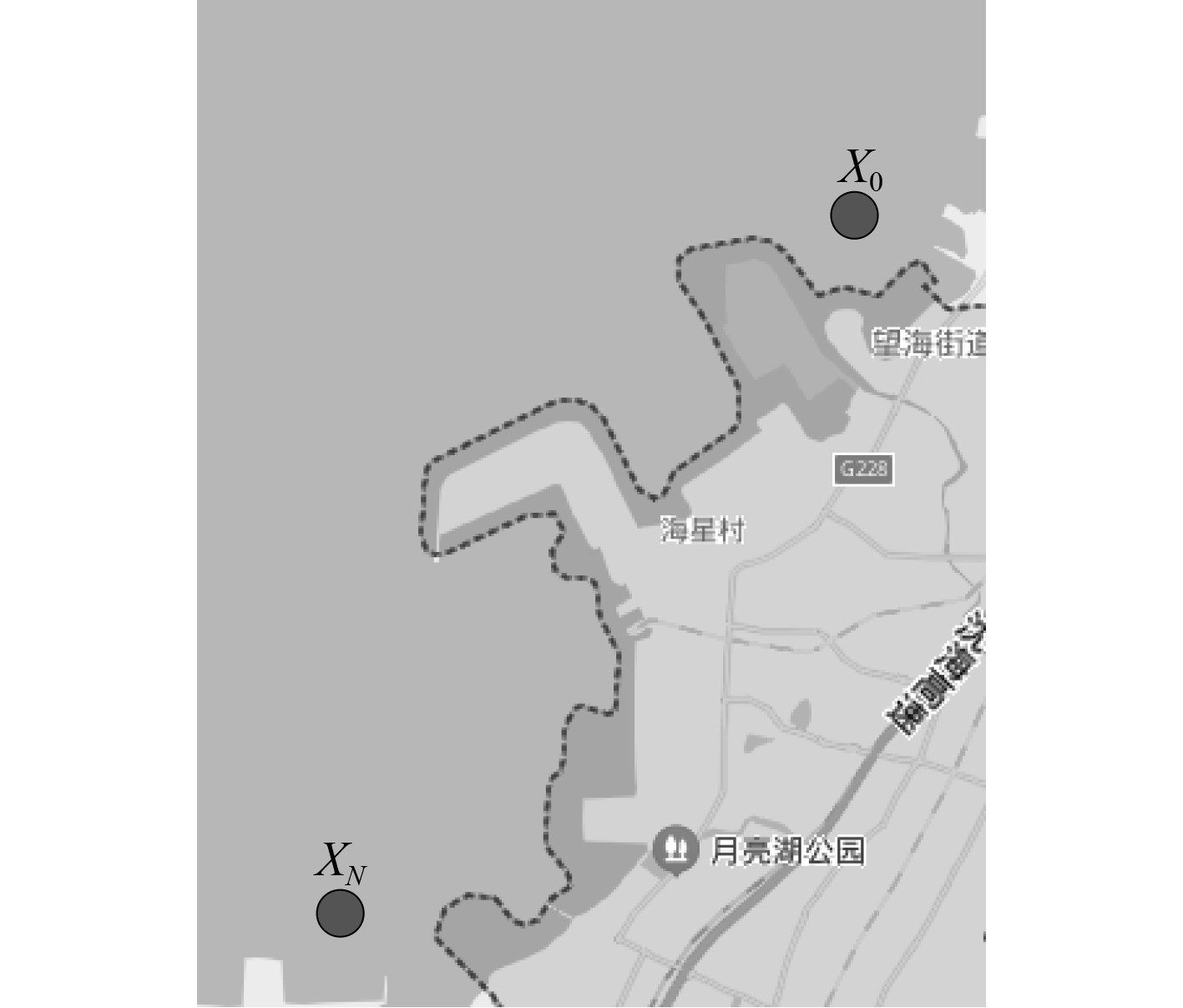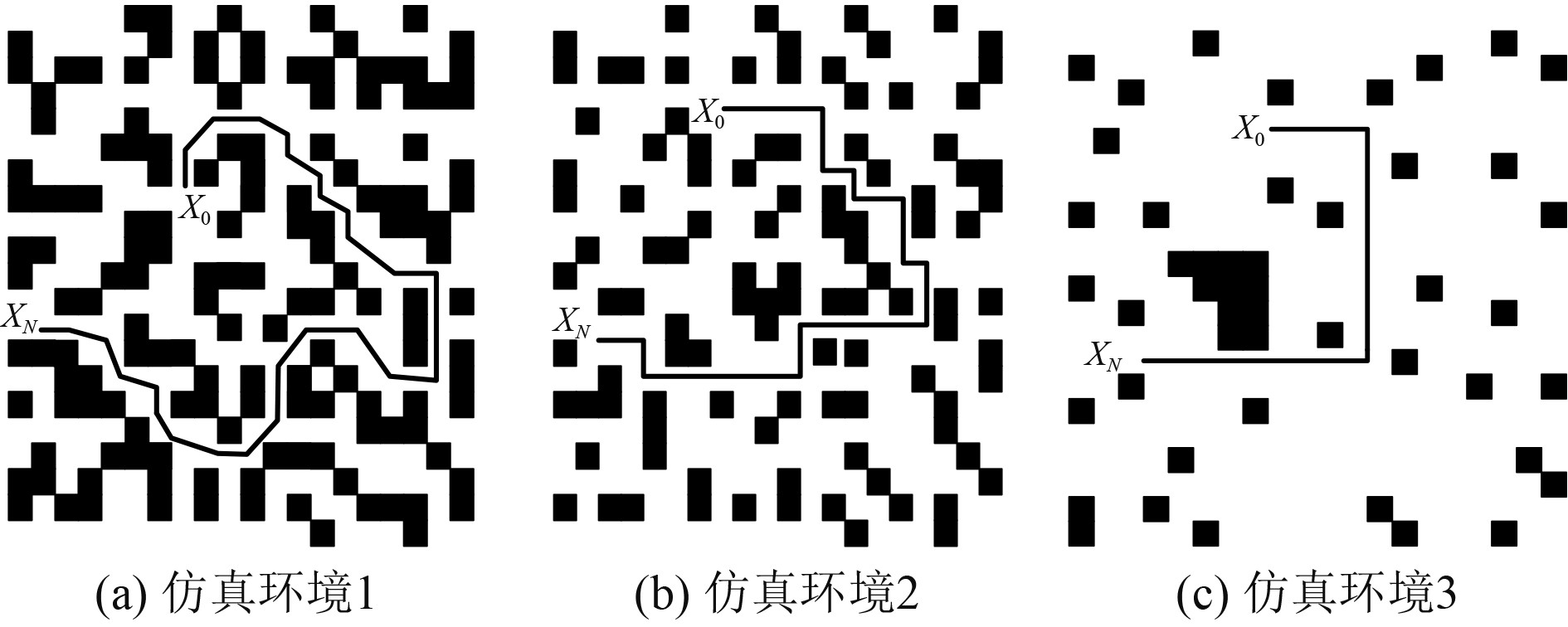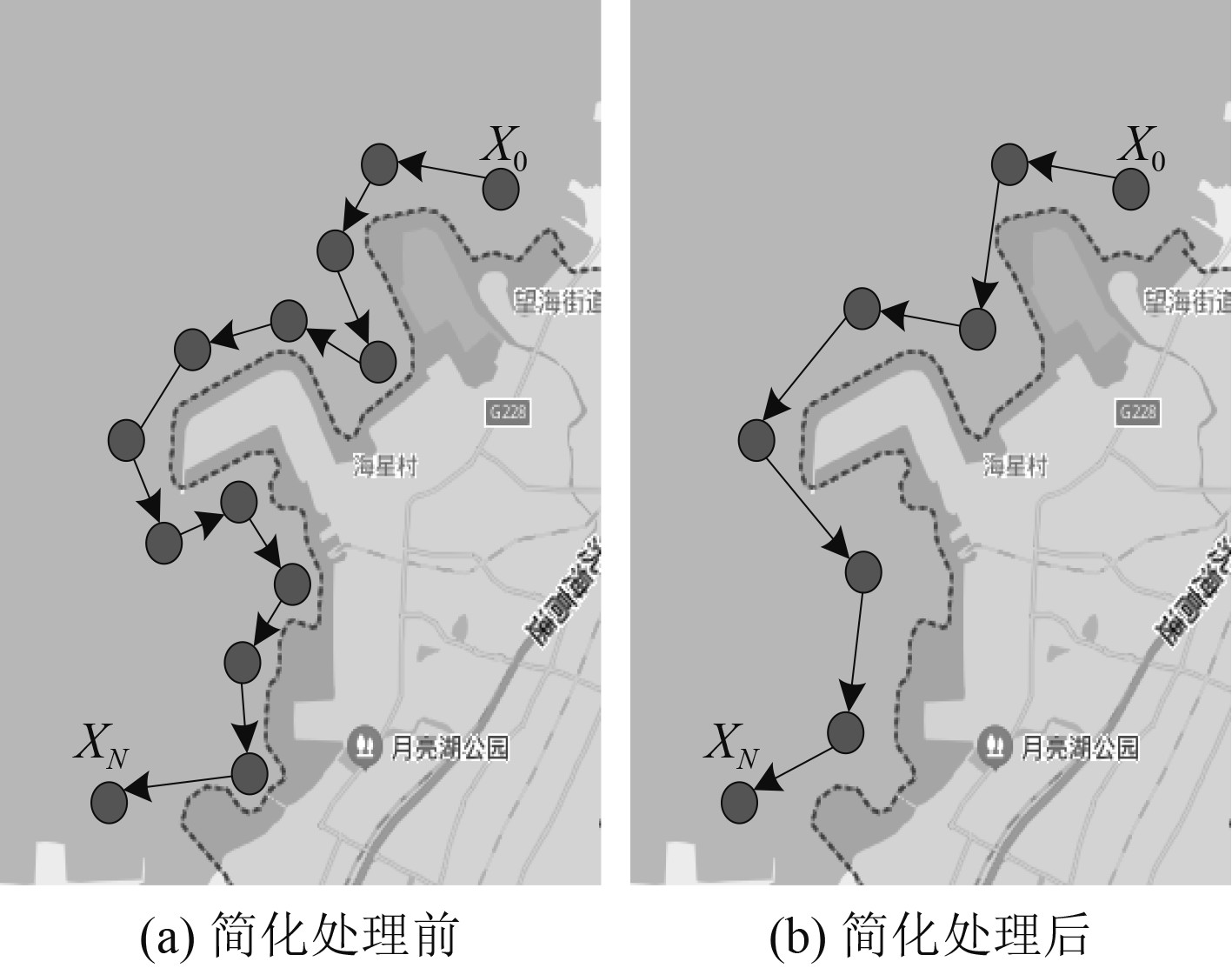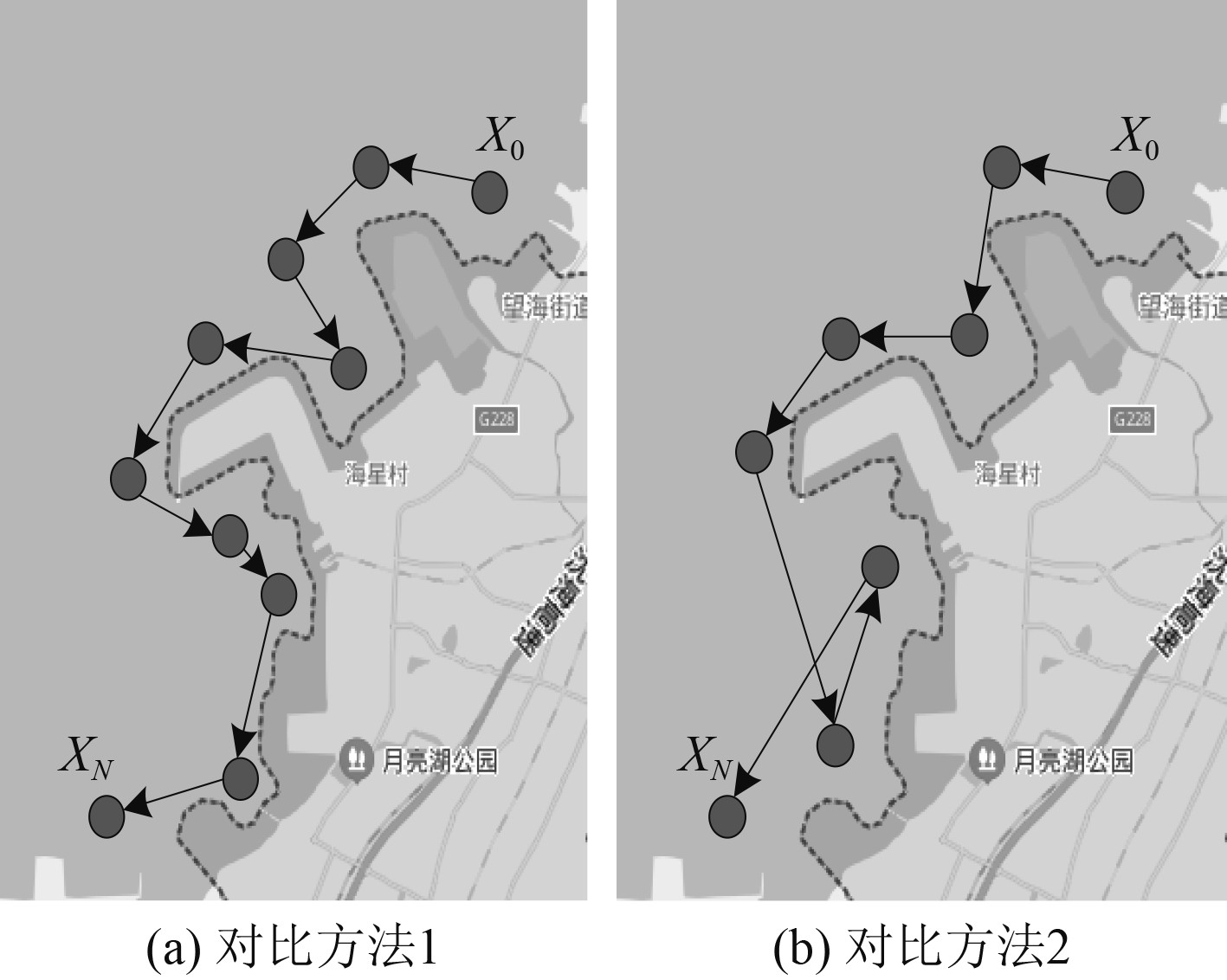﻿ 基于改进A<sup>*</sup>算法的无人船路径规划研究
 舰船科学技术2022, Vol. 44Issue (5): 134-137    DOI: 10.3404/j.issn.1672-7649.2022.05.028PDF

Research on unmanned ship path planning based on improved A* algorithm
LIU Tao
Jiangsu Shipping College, School of Nautical Technology, Nantong 226010, China
Abstract: Aiming at the problems of large memory, long time-consuming and high probability of generating "dead zone" in the process of unmanned ship path planning, an unmanned ship path planning method based on improved A* algorithm is proposed. Select the grid method to build the driving environment model of unmanned ship, use the A* algorithm to determine the cost function, judge the cost, and take the node with the lowest cost as the next track point, so as to obtain the optimal driving path of unmanned ship. In order to improve the A* algorithm, the actual cost function is improved by using the lower limit of the turning radius, the lower limit of the path length and the constraints of the traveling speed conditions of the unmanned ship. The heuristic function is improved by the linear combination of Euclidean distance and Manhattan distance, so as to simplify the navigation path of the unmanned ship, remove the extra nodes and obtain the optimal path. Experimental results show that this method can effectively achieve the purpose of obstacle avoidance, and the path planning time and total voyage are less.
Key words: A * algorithm     unmanned ship     route planning     grid method     cost function     heuristic function
0 引　言

1 改进A*算法的无人船路径规划方法 1.1 基于栅格法的无人船行驶环境模型构建

 $d{\text{ = }}1.5 \times \max \left\{ {{W_c},{W_k}} \right\} 。$ (1)

1.2 基于A*算法的路径规划 1.2.1 A*算法的改进

1）无人船转弯半径下限

 ${R_{\min }} = \frac{v}{u} \times \frac{v}{{\tan {\phi _{\max }}}}。$ (4)

 ${s_R} = \left\{ \begin{gathered} \sum\limits_{k = 1}^n {\frac{1}{{{r_k}}},{r_k} \geqslant {R_{\min }}} , \hfill \\ \infty ,\mathop {}\nolimits_{} {r_k} < {R_{\min }}。\hfill \\ \end{gathered} \right.$ (5)

2）路径长度下限

 ${L_{\min }} = \sqrt {{{\left( {sum{X_0}} \right)}^2} - {{\left( {sum{X_1}} \right)}^2}}，$ (6)

 ${s_L} = \frac{{{L_n}}}{{{L_{\min }}}} \times \varepsilon 。$ (7)

3）行驶速度条件约束

 $0 \leqslant v \leqslant {v_{\max }}。$ (8)

 $\left\{ \begin{gathered} {v_l} = \frac{L}{T} ,\hfill \\ {s_v} = \left| {{v_l} - v} \right|。\hfill \\ \end{gathered} \right.$ (9)

$s\left( x \right)$ 表示考虑无人船转弯半径下限、路径长度下限和行驶速度条件约束的代价函数，其公式描述如下：

 $s\left( {x,y} \right) = \left( {{k_1} \times {s_R} + {k_2} \times {s_L} + {k_3} \times {s_v}} \right) \times \sigma 。$ (10)

 $b\left( {x,y} \right) = m \times \sqrt {{{\left( {N - {X_1}} \right)}^2}} + \left( {1{{ - }}m} \right) \times \left( {\left| {N - {X_1}} \right|} \right)。$ (11)

1.2.2 路径规划流程

1.3 路径规划结果的简化处理

1）设定 $i = 1$ ，在 ${X_p}$ 内添加 ${x_i}$

2）针对各 $j \in \left( {i + 1, \cdots ,N} \right)$ ，确定在点 ${x_i}$ ${x_j}$ 构成的线段 ${x_i}{x_j}$ 间不存在障碍物，在发现第一个障碍物的条件下暂停搜索，同时设定 $i = j - 1$ ，在 ${X_p}$ 内添加 ${x_i}$

3）循环步骤过程至 $i = N$ 为止，由此获取简化后的无人船航迹路径。

2 实验结果与分析图 1 实验对象航行区域图 Fig. 1 Navigation area of experimental object
2.1 有效性验证

1）在实验对象处于图2(a)所示的仿真环境内，实验对象航行区域内的障碍物较多，同时实验对象由X0XN的转弯行驶次数高于直行次数，m的取值范围为[0,0.35]；图 2 m值取值标准 Fig. 2 m value standard

2）在实验对象处于图2(b)所示的仿真环境内，实验对象航行区域内的障碍物数量适中，同时实验对象由X0XN的转弯行驶次数与直行次数约一致，m的取值范围为[0.35,0.70]；

3）在实验对象处于图2(c)所示的仿真环境内，实验对象航行区域内的障碍物数量较少，同时实验对象由X0XN的转弯行驶次数低于直行次数，m的取值范围为[0.70,1.00]。

2.2 实际路径规划结果图 3 本文方法路径规划结果 Fig. 3 Path planning results of this method图 4 对比方法路径规划结果 Fig. 4 Comparison method and path planning results表 1 本文方法与对比方法路径规划性能对比 Tab.1 Comparison of path planning performance between this method and comparison method
3 结　语

  吕太之, 张军, 陈勇. 一种基于流式计算的无人船路径规划算法[J]. 船舶工程, 2021, 43(S1): 348-352.  龚波, 周永华, 艾矫燕. 融合卡尔曼滤波的无人船航向神经网络控制[J]. 计算机工程与设计, 2020, 41(8): 2315-2320.  赵红, 赵德润, 王宁, 等. 改进型BINN算法应用在无人船优先区域覆盖路径规划的研究[J]. 中国造船, 2020, 61(2): 91-102. DOI:10.3969/j.issn.1000-4882.2020.02.009  刘蔚, 谈果戈, 邹劲, 等. 基于快速行进平方法的水面无人船路径规划[J]. 信息与控制, 2021, 50(3): 308-320.  乔双虎, 郑凯, 陈亚博. 一种基于拓展支持向量机的无人船路径规划方法[J]. 船舶工程, 2020, 42(7): 130-137.  胡锟, 张亮. 罚函数凸优化迭代算法及在无人机路径规划中的应用[J]. 计算机应用研究, 2021, 38(3): 725-728.  王宇, 王文浩, 徐凡, 等. 基于改进蚁群算法的植保无人机路径规划方法[J]. 农业机械学报, 2020, 51(11): 103-112+92. DOI:10.6041/j.issn.1000-1298.2020.11.011  祁玄玄, 黄家骏, 曹建安. 基于改进A^*算法的无人车路径规划[J]. 计算机应用, 2020, 40(7): 2021-2027.  高峰, 周浩, 杨卓宇. 基于改进A^*算法的水面无人船全局路径规划[J]. 计算机应用研究, 2020, 37(S1): 120-121+125.  曹莹, 陈沿伊, 冯睿. 基于改进的A^*算法集装箱码头自动导引小车路径规划研究[J]. 武汉理工大学学报:交通科学与工程版, 2020, 44(4): 738-742.